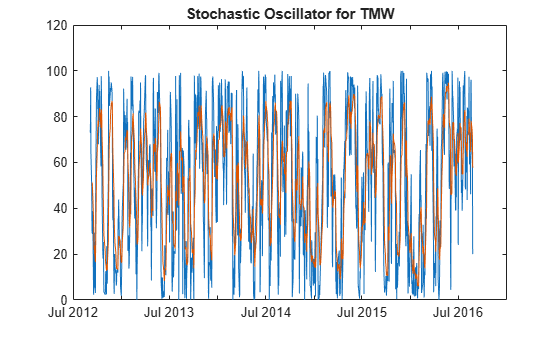# stochosc

Stochastic oscillator

`stochosc` has been partially removed and will no longer accept a `fints` object (`tsobj`) argument. Use a matrix, `timetable`, or `table` instead for financial time series.

Use `fts2timetable` to convert a `fints` object to a `timetable` object.

## Syntax

``percentKnD = stochosc(Data)``
``percentKnD = stochosc(___,Name,Value)``

## Description

example

````percentKnD = stochosc(Data)` calculates the stochastic oscillator.```

example

````percentKnD = stochosc(___,Name,Value)` adds optional name-value pair arguments. ```

## Input Arguments

collapse all

Data with high, low, open, close information, specified as a matrix, table, or timetable. For matrix input, `Data` is an `M`-by-`3` matrix of high, low, and closing prices stored in the corresponding columns, respectively. Timetables and tables with `M` rows must contain variables named `'High'`, `'Low'`, and `'Close'` (case insensitive).

Data Types: `double` | `table` | `timetable`

### Name-Value Pair Arguments

Specify optional comma-separated pairs of `Name,Value` arguments. `Name` is the argument name and `Value` is the corresponding value. `Name` must appear inside quotes. You can specify several name and value pair arguments in any order as `Name1,Value1,...,NameN,ValueN`.

Example: ```percentKnD = stochosc(TMW,'NumPeriodsD',10,'NumPeriodsK',3,'Type','exponential')```

Period difference for PercentK, specified as the comma-separated pair consisting of `'NumPeriodsK'` and a scalar positive integer.

Data Types: `double`

Length of moving average in periods for PercentD, specified as the comma-separated pair consisting of `'NumPeriodsD'` and a scalar positive integer.

Data Types: `double`

Moving average method for PercentD calculation, specified as the comma-separated pair consisting of `'Type'` and a character vector with a value of:

• `'exponential'` – Exponential moving average is a weighted moving average. Exponential moving averages reduce the lag by applying more weight to recent prices. For example, a 10 period exponential moving average weights the most recent price by 18.18%.

• `'triangular'` – Triangular moving average is a double-smoothing of the data. The first simple moving average is calculated and then a second simple moving average is calculated on the first moving average with the same window size.

Data Types: `char`

## Output Arguments

collapse all

PercentK and PercentD, returned with the same number of rows (`M`) and type (matrix, table, or timetable) as the input `Data`.

## Examples

collapse all

Load the file `SimulatedStock.mat`, which provides a timetable (`TMW`) for financial data for TMW stock.

```load SimulatedStock.mat oscillator = stochosc(TMW,'NumPeriodsD',7,'NumPeriodsK',10,'Type','exponential'); plot(oscillator.Time,oscillator.FastPercentK,oscillator.Time,oscillator.FastPercentD) title('Stochastic Oscillator for TMW')```collapse all

### Stochastic Oscillator

The stochastic oscillator calculates the Fast PercentK (F%K), Fast PercentD (F%D), Slow PercentK (S%K), and Slow PercentD (S%D) from the series of high, low, and closing stock prices.

By default, the stochastic oscillator is based on 10-period difference for PercentK and a 3-period exponential moving average for PercentD.

 Achelis, S. B. Technical Analysis from A to Z. Second Edition. McGraw-Hill, 1995, pp. 268–271.